## Pebble Fonts and Metar Watchface6 comments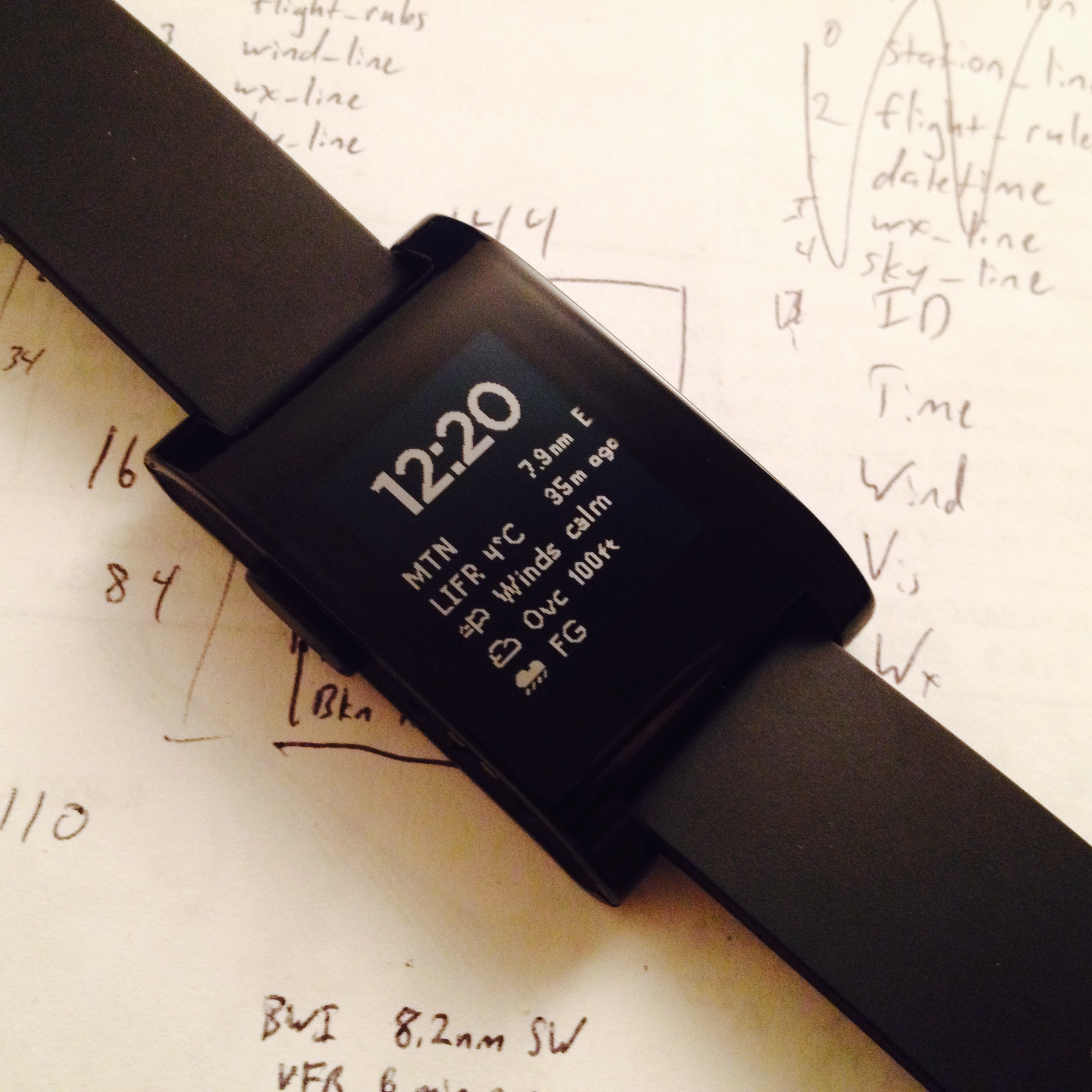For Christmas, my brother gave me a Pebble smart watch. In addition to showing the time in a variety of ways, this little watch can connect to a smartphone over Bluetooth to show text messages and caller ID information. It also interfaces with a variety of sports tracking apps, including my favorite, iSmoothRun. The most exciting feature of the Pebble, though, is that it’s an open platform with a simple development kit for the C language and streamlined developer’s tools.

The holidays came in the midst of the Pebble SDK 2.0 release, which includes a JavaScript environment allowing watch apps to make use of the phone’s internet connection and GPS signal. One of the most popular applications of the Pebble-phone connection is to display the local weather in addition to the time. Partly as an exercise to learn the Pebble system and partly to create a handy way to check aviation weather, I wrote a watchface that displays the nearest METAR — a standardized aviation forecast — alongside the time.

The project involved a PHP backend, C watch app, JavaScript phone component, and — perhaps most surprisingly — becoming intimately acquainted with the Pebble font resource file format. The lion’s share of this post describes generating a custom font for Pebble; the rest gives an overview of the Metar watchface itself.

# First Steps Toward a Custom Pebble Font

It’s an idea older than Wingdings and score lines on 8-bit games: Sometimes the easiest way to display a simple graphic, especially one that will be intermingled with text, is to map the graphic to a character in a custom font. This strategy is ideal for the Metar watchface: mapping symbols (eg for cloud cover) to characters not only simplifies alignment with text, but also provides an easy way for a PHP backend to output the semi-graphical forecast.

The Pebble firmware supplies a number of attractive fonts and API functions to access both built-in and custom font resources. The SDK also includes `Pebble/tools/font/fontgen.py`, a Python script that rasterizes a TrueType font at a given size, outputting a ready-for-Pebble `.pfo` file. This isn’t a script you have any reason to run yourself: As the included `feature-custom-font` example demonstrates, the Pebble build tools will run it automatically if your `.ttf` and `appinfo.json` files are appropriately configured.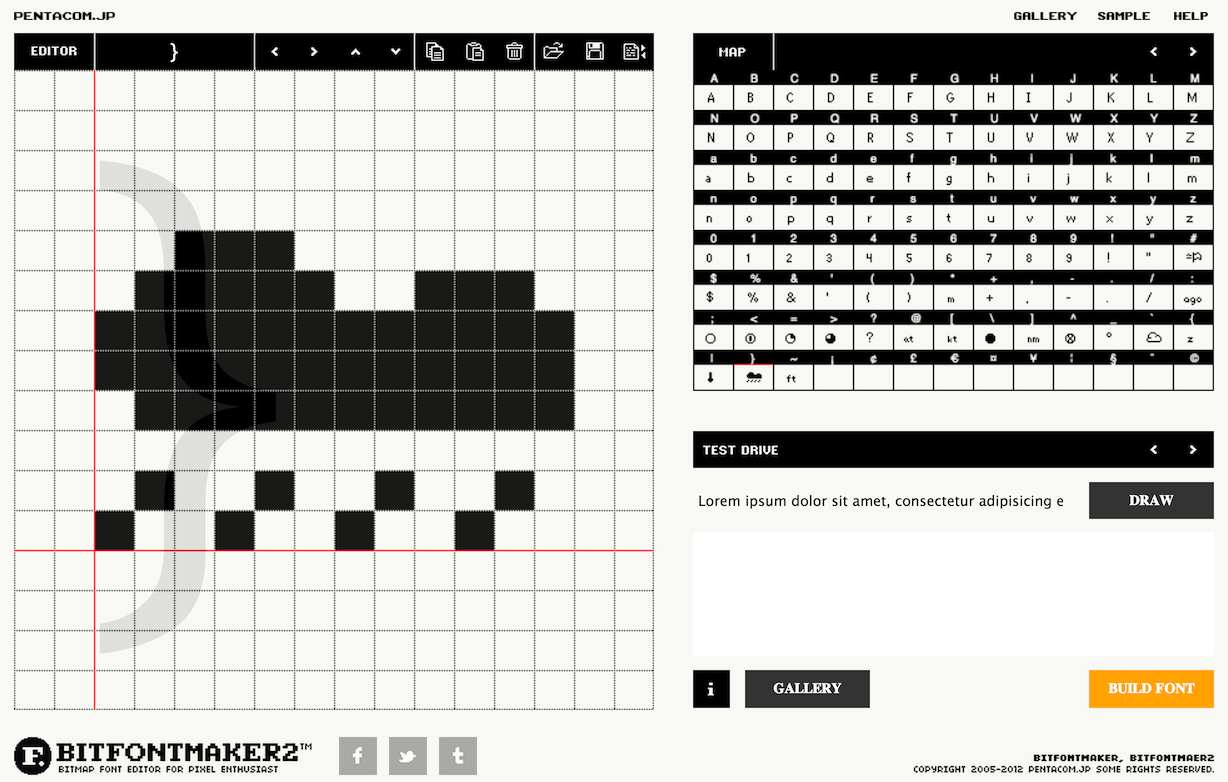The question, then, is how to make your `.ttf` file. If you’re a professional designer, you probably have one of the very expensive font packages lying around. If you’re like the rest of us, you’re probably looking for a free font creation tool. I went with BitFontMaker (pictured) because at the small character sizes I’m working with, the annoyance of drawing splines or even defining corner behavior seems to be more trouble than it’s worth. BitFontMaker also includes the “map” view to remind you which of your symbols is mapped to which character.

Although the next section describes in detail one method to edit the font pixel-by-pixel, I should point out that in my experience, just converting the `.ttf` from BitFontMaker using the standard Pebble workflow was very close to what I was looking for. The photo at the beginning of this post was taken before any refinement.

# The Pebble Font Format

Although the Pebble font automatically generated from the `.ttf` file was pretty good, any small binary image — including these characters — will look better when refined by hand. And to do that, we need direct access to the Pebble font data. As mentioned above, the Pebble SDK’s `Pebble/tools/font/fontgen.py` describes the `.pfo` font resource file, and the PebbleDev Wiki has a page on the format as well.

```#!/usr/bin/env python

import argparse
import freetype
import os
import re
import struct
import sys
import itertools
from math import ceil

# Font
#   FontInfo
#       (uint8_t)  version
#       (uint8_t)  max_height
#       (uint16_t) number_of_glyphs
#   (uint32_t) offset_table[]
#       this table contains offsets into the glyph_table for characters 0x20 to 0xff
#       each offset is counted in 32-bit blocks from the start of the glyph
#       table. 16-bit offsets are keyed by 16-bit codepoints.
#       packed:     (uint_16) codepoint
#                   (uint_16) offset
#
#   (uint32_t) glyph_table[]
#       : the 32-bit block for offset 0 is used to indicate that a glyph is not supported
#       then for each glyph:
#       [offset + 0]  packed:   (int_8) offset_top
#                               (int_8) offset_left,
#                              (uint_8) bitmap_height,
#                              (uint_8) bitmap_width (LSB)
#
#       [offset + 1]           (int_8) horizontal_advance
#         [offset + 2] bitmap data (unaligned rows of bits), padded with 0's at
#         the end to make the bitmap data as a whole use multiples of 32-bit
#         blocks

MIN_CODEPOINT = 0x20
MAX_CODEPOINT = 0xffff
```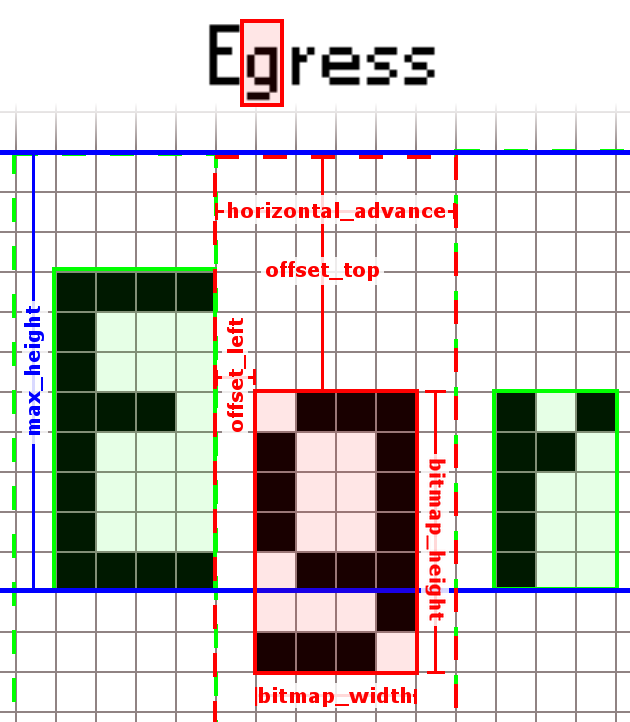A bit of trial and error revealed the meaning of each of these parameters. The font file begins with three values defining the font as a whole: a version number, the nominal height above baseline, and the number of glyphs in the font. This is followed by a lookup table character, encoding each character’s unicode value (two bytes) and the memory offset to its bitmap information. The main data block follows. For each character, it stores, in order:

• `offset_top`, the distance from the font’s top-line to the top of the bitmap
• `offset_left`, the distance from the right edge of the preceding character to the left edge of the bitmap
• `bitmap_height` and `bitmap_width`, which are needed to decode the bitmap
• `horizontal_advance`, the total horizontal space the character takes up, including left and right padding, and
• the bitmap information itself, stored in a multiple of 8 bytes

The metrics are shown graphically to the right. In general, the horizontal advance is the sum of the left offset, the bitmap width, and the (unspecified) right offset. The descent of the character below the baseline is equal to the top offset plus the bitmap height minus the font’s nominal height.

# Making it Pixel-Perfect

There is an interesting git repository for working with Pebble fonts, and if you look hard enough you can find a few other resources online, but most activity on this front involves adding non-Latin characters to custom Pebble firmware. After playing with the tools online, I decided to write a fresh Python script that could read a `.pfo` file and manipulate the font more easily in code. Additionally, the script allows exporting the font into a standard `.png` image. After the image is modified (using any editor), the script can read it back in and produce a corrected `.pfo` file for use in a Pebble app.

This script functions at two levels: As a Python module, it can be used directly through an interactive Python session or imported into your own program to handle the font backend. As a standalone command line utility, it can perform the most common functions in a self-documenting way. The basic class is `PebbleFontFile`, which has methods for reading and writing `.pfo` and `.png` files as well as accessing individual characters. Characters are stored as instances of the `PebbleFontCharacter` class, which exposes the bitmap as a 2-d boolean NumPy array. The program requires Python 2.5 or later, NumPy, and bitstring, and the Python Imaging Library, all of which can be installed with pip. You can peruse below, or download it.

One important quirk of my `PebbleFontCharacter` is that it abstracts away the `horizontal_advance` characteristic of the characters, instead using `lmargin` and `rmargin` variables as the left and right margins, respectively. The advantage is that you can change the width of a glyph without recalculating the spacing. (The bitmap dimensions are read directly from the glyph array’s shape.) The disadvantage is that it’s less intuitive, for instance, to generate an acute accent character with an advance less than the character width. (I’m not sure whether this would cause errors in the Pebble text display system.) Although it’s less intuitive, a negative value for `rmargin` would do the trick.

```# pebbleFonts.py v1.0
# John McManigle, 17 Feb 2014
#
# but you are free to use this for any purpose.  No warranty, express or implied.

import argparse
import pickle
import itertools
import numpy as np
from struct import pack,unpack
from bitstring import BitArray
import sys
from PIL import Image

class PebbleFontCharacter(object):
def __init__(self, c):
self.c = unichr(c)
self.lmargin = 0
self.rmargin = 0
self.tmargin = 0
self.u1 = 0
self.u2 = 0
self.u3 = 0
self.bitarray = None

def __str__(self):
s = 'pebbleFonts.PebbleFontCharacter \''+self.c.encode('utf-8')+'\'\n'
s += '  L-margin='+str(self.lmargin)+';'
s += '  R-margin='+str(self.rmargin)+';'
s += '  T-margin='+str(self.tmargin)+';'
if self.bitarray != None:
s += '  W='+str(self.bitarray.shape)+';'
s += '  H='+str(self.bitarray.shape)+''

ya = 0
for line in self.bitarray:
s += '\n   |'
for char in line:
if char: s += 'X'
else:    s += ' '
s += '|'
else: s += ' (No bit array.)'
return s

def setMeta(self, data):
(  width,  height, self.lmargin, self.tmargin,
self.u1, self.u2,      self.u3,      spacing ) = unpack("2B2b3Bb", data)
self.rmargin = spacing - self.lmargin - width
return (width, height)

def setBits(self, data, width, height):
bits = BitArray(bytes=data)
array = np.array(bits)
for i in range(0, len(array), 8):
array[i:i+8] = array[i:i+8][::-1]
self.bitarray = np.reshape(array[:width*height], (height,width))

def width(self):
if self.bitarray == None: return 0
return self.bitarray.shape

def height(self):
if self.bitarray == None: return 0
return self.bitarray.shape

def packed(self):
if self.bitarray != None: h,w = self.bitarray.shape
else:                     h,w = 0,0

spacing = self.lmargin + w + self.rmargin
s = pack("2B2b3Bb", w, h, self.lmargin, self.tmargin, self.u1, self.u2, self.u3, spacing)

if self.bitarray != None:
array = self.bitarray.flatten().tolist()
r = len(array) % 32
if r: array.extend([False] * (32-r))
array = np.asarray(array)
for i in range(0, len(array), 8):
array[i:i+8] = array[i:i+8][::-1]
bytes = BitArray(bin=''.join('1' if b else '0' for b in array))
s += bytes.bytes

return s

def bit_array_packed_size(self):
if self.bitarray != None:
return int(np.ceil(self.bitarray.size/32.0))
else: return 0

def trimArray(self):
if self.bitarray == None: return
while not np.any(self.bitarray[0,:]):
self.bitarray = np.delete(self.bitarray, 0, 0)
self.tmargin += 1
while not np.any(self.bitarray[-1,:]):
self.bitarray = np.delete(self.bitarray, -1, 0)
while not np.any(self.bitarray[:,0]):
self.bitarray = np.delete(self.bitarray, 0, 1)
while not np.any(self.bitarray[:,-1]):
self.bitarray = np.delete(self.bitarray, -1, 1)

class PebbleFontFile(object):

def __init__(self, filename=None):
self.char_table = {}
if filename != None:

def __len__(self):
return len(self.char_table)

def __getitem__(self, key):
return self.char_table[ord(key)]

def __setitem__(self, key, value):
self.char_table[ord(key)] = value

def __delitem__(self, key):
del(self.char_table[ord(key)])

def __iter__(self):
return self.iterkeys()

def iterkeys(self):
return itertools.imap(unichr, self.char_table.iterkeys())

def __str__(self):
s = 'pebbleFonts.PebbleFontFile ('+self.orig_filename+')\n'
s += '  '+str(len(self))+' Characters'
return s

self.orig_filename = filename

with open(filename,'rb') as inf:

self.version, self.nom_height, num_entries, self.failsafe_char \
= unpack("2B2H", data[:6])

lookup_table = {}
for i in range(num_entries):
char, offset = unpack("2H", data[6+i*4:10+i*4])
lookup_table[char] = offset

data_offset = 6 + num_entries * 4

for c in lookup_table:
offset = lookup_table[c]*4 + data_offset
char = PebbleFontCharacter(c)
w, h = char.setMeta(data[offset:offset+8])

if( w * h > 0 ):
offset += 8
length = int(np.ceil(w*h/8.0))
char.setBits(data[offset:offset+length], w, h)

self[unichr(c)] = char

def save(self, filename = None):
if filename is None:
filename = self.orig_filename

with open(filename, 'wb') as file:
file.write(pack("2B2H", self.version, self.nom_height, len(self), self.failsafe_char))

offset = 1
for c in sorted(self.iterkeys()):
file.write(pack("2H", ord(c), offset))
offset += 2 + self[c].bit_array_packed_size()

file.write(pack("2H", 0, 0))

for c in sorted(self.iterkeys()):
file.write( self[c].packed() )

file.close()

def saveImage(self, filename):
tot_width = np.amax([self[x].width() for x in self]) + 4
tot_height = np.sum([self[x].height() for x in self]) + len(self)
img = Image.new('1',(tot_width,tot_height),0)

y = 0
for c in sorted(self.iterkeys()):
if self[c].bitarray != None:
ya = 0
for l in self[c].bitarray:
x = 0
if (ya + 1 == self.nom_height - self[c].tmargin):
pix[x, y] = 1
x += 2
for p in l:
pix[x, y] = p
x += 1
if (ya + 1 == self.nom_height - self[c].tmargin):
pix[x+1, y] = 1
y += 1
ya += 1
y += 1
img.save(filename,'PNG')

img = Image.open(filename)

y = 0
for c in sorted(self.iterkeys()):
if self[c].bitarray != None:
for l in range(self[c].bitarray.shape):
x = 2
for p in range(self[c].bitarray.shape):
self[c].bitarray[l,p] = pix[x,y]
x += 1
y += 1
y += 1

def fixWidths(self):
for c in self.iterkeys():
if self[c].bitarray != None:
if self[c].lmargin > 1:
self[c].lmargin = 1
if self[c].rmargin > 1:
self[c].rmargin = 1

for c in self.iterkeys():
if self[c].bitarray != None:
self[c].bitarray = np.pad(self[c].bitarray, p, 'constant', constant_values=False)
self[c].tmargin -= p
self.sanitizeHeight()

def trimAllChars(self):
for c in self.iterkeys():
self[c].trimArray()
self.sanitizeHeight()

def sanitizeHeight(self):
min_t = min(self[c].tmargin for c in self.iterkeys())
self.nom_height -= min_t

for c in self.iterkeys():
self[c].tmargin -= min_t

def printAllChars(self):
for c in sorted(self.iterkeys()):
print self[c],'\n'

if __name__ == '__main__':
parser = argparse.ArgumentParser(
description='Performs operations on a Pebble font file (.pfo).  Uses a scratch file (.pkl) to store intermediate steps, so this command can be used sequentially for multiple operations.  A typical use case is to --loadPFO to get the font into working memory.  Then, save as a PNG image with --savePNG and edit in an external editor before loading the modified image back into working memory with --loadPNG.  Finally, use --savePFO to update the Pebble font file and put it in your Pebble app.  This is an interface over a fairly versatile Python class, so if you don\'t see what you need in the options here, import it into your own Python script and go nuts.  Do back up your .pfo file before you start.',
epilog='pebbleFonts.py v1.0, by John McManigle, 17 Feb 2014.  Use freely.'
)
help='Use specified scratch file. (default: font.pkl)')
help='Use specified .png file for --savePNG or --loadPNG (default: font.png)')
action_group = parser.add_argument_group('Action', 'Select one or more actions from the following options. Each action may only be taken once per run, and they will always be executed in the order in which they appear below.')
help='Load new working font from a .pfo file.')
help='Print a character from the working font to screen.')
help='Print all characters from the working font to screen.')
help='Output font to .png image, padding each character by [pad] pixels. (default: 3) (see -i above)')
help='Save .pfo file. (default: overwrite loaded .pfo file)')

if len(sys.argv) > 1: args = parser.parse_args()
else:                 args = parser.parse_args(['-h'])

try:
pkl_file = open(args.t, 'rb')
except IOError:
print 'Error: Could not find scratch file.  Did you forget to --loadPFO?'
sys.exit()
else:
pkl_file.close()
else:

if args.printChar is not None:
print ff[args.printChar]

if args.printAllChars is True:
ff.printAllChars()

if args.savePNG is not None:
print 'Padding each character with',args.savePNG,'pixel margins'
print 'Saving composite font image to',args.i
ff.saveImage(args.i)

print 'Trimming extra padding from each character'
ff.trimAllChars()

if args.sanitize is True:
print 'Trimming extra padding from each character'
ff.trimAllChars()
print 'Sanitizing left and right margins'
ff.fixWidths()

if args.savePFO is not None:
if args.savePFO is True:
print 'Saving font to Pebble file',ff.orig_filename
ff.save()
else:
print 'Saving font to Pebble file',args.savePFO
ff.save(args.savePFO)

print 'Saving font to scratch file',args.t
with open(args.t, 'wb') as pkl_file:
pickle.dump(ff, pkl_file)```

The little program did the trick for me, and I hope it’s as useful for some other Pebble programmers out there. The completed `.pfo` file, for those interested, is here.

# The Metar Watchface

In comparison to the above, the programming behind the Metar Watchface is fairly simple. The system has three components. The first is a PHP script which takes a given latitude and longitude and returns a text representation of the nearest METAR. It takes advantage of NOAA’s Aviation Digital Data Service for timely weather information, and its spatial calculations borrow heavily from Chris Veness’s JavaScript algorithms. In short, the script will:

1. Retrieve a list of METAR reporting sites known to NOAA.
2. Find the nearest site to the given lat/lon with a valid METAR.
3. Decode the METAR and convert it to a pretty format using characters from the Pebble font above.
```<?php
/* nearestmetar.php v1.0, John McManigle, 17 Feb 2014
* but you are free to use this for any purpose.  No warranties, express or implied.
* Not for flight planning or navigation.
*
* Latitude/longitude formulae & scripts adapted from Chris Veness's JavaScript
* versions at http://www.movable-type.co.uk/scripts/latlong.html
*/

function compass_mod(\$x)
{
return fmod(\$x+360.0,360.0);
}

function compass_direction(\$x)
{
switch( intval(floor(compass_mod(\$x)/22.5)) ) {
case 0:
return 'N';
case 1:
case 2:
return 'NE';
case 3:
case 4:
return 'E';
case 5:
case 6:
return 'SE';
case 7:
case 8:
return 'S';
case 9:
case 10:
return 'SW';
case 11:
case 12:
return 'W';
case 13:
case 14:
return 'NW';
case 15:
return 'N';
}
return 'Error';
}

function latlon_to_decdeg(\$dmsStr) {

if (is_numeric(\$dmsStr)) return floatval(\$dmsStr);

\$dms = trim(\$dmsStr);
\$dms = preg_replace('/^-/','',\$dms);
\$dms = preg_replace('/[NSEW]\$/i','',\$dms);
\$dms = preg_split('/[^0-9.,]+/',\$dms);
if (\$dms[sizeof(\$dms)-1]=='') array_pop(\$dms);  // from trailing symbol

if (\$dms == '') return NULL;

switch (sizeof(\$dms)) {
case 3:  // interpret 3-part result as d/m/s
\$deg = \$dms/1 + \$dms/60 + \$dms/3600;
break;
case 2:  // interpret 2-part result as d/m
\$deg = \$dms/1 + \$dms/60;
break;
case 1:  // just d (possibly decimal) or non-separated dddmmss
\$deg = \$dms;
break;
default:
return NaN;
}

if (preg_match('/^-|[WS]\$/i',trim(\$dmsStr))) \$deg = -\$deg; // take '-', west and south as -ve
return floatval(\$deg);
}

function latlon_distance_nm(\$lat_a, \$lon_a, \$lat_b, \$lon_b)
{
\$r = 6371; // km

\$a = sin(\$dLat/2) * sin(\$dLat/2) + sin(\$dLon/2) * sin(\$dLon/2) * cos(\$lat_a) * cos(\$lat_b);
\$c = 2 * atan2(sqrt(\$a), sqrt(1-\$a));
\$d = \$r * \$c;

return (0.539957 * \$d); // nm
}

function latlon_init_true_bearing(\$lat_a, \$lon_a, \$lat_b, \$lon_b)
{

\$y = sin(\$dLon) * cos(\$lat_b);
\$x = cos(\$lat_a) * sin(\$lat_b) - sin(\$lat_a) * cos(\$lat_b) * cos(\$dLon);

return \$brng;
}

function latlon_magnetic_declination(\$lat, \$lon)
{
\$csv = file_get_contents('http://www.ngdc.noaa.gov/geomag-web/calculators/calculateDeclination?lat1='.\$lat.'&lon1='.\$lon.'&resultFormat=csv');
\$line = explode("\n", \$csv);
\$field = explode(',',\$line);
return \$field;
}

function latlon_init_mag_bearing(\$lat_a, \$lon_a, \$lat_b, \$lon_b)
{
\$true = latlon_init_true_bearing(\$lat_a, \$lon_a, \$lat_b, \$lon_b);
\$declination = latlon_magnetic_declination(\$lat_a, \$lon_a);
return compass_mod(\$true - \$declination);
}

function parse_station(\$line)
{
if(strlen(\$line) != 83) return NULL;
\$station = [];
\$station['cd'] = substr(\$line, 0, 2);
\$station['station'] = trim(substr(\$line, 3, 16));
\$station['icao'] = substr(\$line, 20, 4);
\$station['iata'] = substr(\$line, 26, 3) == '   ' ? NULL : substr(\$line, 26, 3);
\$station['synp'] = substr(\$line, 32, 5) == '     ' ? NULL : intval(substr(\$line, 32, 5));
\$station['lat'] = substr(\$line, 39, 6);
\$station['lon'] = substr(\$line, 47, 7);
\$station['elev'] = intval(trim(substr(\$line, 55, 4)));
\$station['m'] = \$line;
\$station['n'] = \$line;
\$station['v'] = \$line;
\$station['u'] = \$line;
\$station['a'] = \$line;
\$station['c'] = \$line;
\$station['priority'] = intval(\$line);
\$station['country'] = substr(\$line, 81, 2);

\$station['metar'] = (\$station['m'] == 'X');
\$station['declat'] = latlon_to_decdeg(\$station['lat']);
\$station['declon'] = latlon_to_decdeg(\$station['lon']);

return \$station;
}

function get_nearest_stations(\$lat, \$lon)
{
\$stations = explode("\n", \$stations);

\$nearest_pairs = [];

foreach( \$stations as \$line )
{
if(strlen(\$line) != 83) continue;
if(\$line != 'X')    continue;
\$station = parse_station(\$line);
\$dist = latlon_distance_nm(\$lat,\$lon,\$station['declat'],\$station['declon']);

if( sizeof(\$nearest_pairs) < 3 || \$dist < \$nearest_pairs['distance'])
{
\$pair = [];
\$pair['distance'] = \$dist;
\$pair['station'] = \$station;
\$nearest_pairs[] = \$pair;
usort(\$nearest_pairs, function(\$a,\$b){return \$a['distance']-\$b['distance'];});
if(sizeof(\$nearest_pairs) > 3)
unset(\$nearest_pairs);
}
}

return \$nearest_pairs;
}

function get_metar(\$icao)
{
\$line = explode("\n",file_get_contents(\$url));
\$fields = explode(',',\$line);

\$metar = [];
\$metar['raw_text']              = \$fields;
\$metar['station_id']            = \$fields;
\$metar['observation_time']      = \$fields;
\$metar['latitude']              = \$fields == '' ? NULL : floatval(\$fields);
\$metar['longitude']             = \$fields == '' ? NULL : floatval(\$fields);
\$metar['temp_c']                = \$fields == '' ? NULL : floatval(\$fields);
\$metar['dewpoint_c']            = \$fields == '' ? NULL : floatval(\$fields);
\$metar['wind_dir_degrees']      = \$fields == '' ? NULL : intval(\$fields);
\$metar['wind_speed_kt']         = \$fields == '' ? NULL : intval(\$fields);
\$metar['wind_gust_kt']          = \$fields == '' ? NULL : intval(\$fields);
\$metar['visibility_statute_mi'] = \$fields == '' ? NULL : floatval(\$fields);
\$metar['altim_in_hg']           = \$fields == '' ? NULL : floatval(\$fields);
\$metar['sea_level_pressure_mb'] = \$fields == '' ? NULL : floatval(\$fields);
\$metar['corrected']             = (bool)\$fields;
\$metar['auto']                  = (bool)\$fields;
\$metar['auto_station']          = (bool)\$fields;
\$metar['maintenance_indicator_on'] = (bool)\$fields;
\$metar['no_signal']             = (bool)\$fields;
\$metar['lightning_sensor_off']  = (bool)\$fields;
\$metar['freezing_rain_sensor_off'] = (bool)\$fields;
\$metar['present_weather_sensor_off'] = (bool)\$fields;
\$metar['wx_string']             = \$fields;

\$metar['sky_cover'] = [];
foreach(range(0,3) as \$n)
{
if(\$fields[22+2*\$n] != '')
{
\$metar['sky_cover'][\$n] = [];
\$metar['sky_cover'][\$n]['sky_cover'] = \$fields[22+2*\$n];
\$metar['sky_cover'][\$n]['cloud_base_ft_agl'] = \$fields[22+2*\$n+1] == '' ? NULL : intval(\$fields[22+2*\$n+1]);
}
}

\$metar['flight_category'] = \$fields;
\$metar['three_hr_pressure_tendency_mb'] = \$fields == '' ? NULL : floatval(\$fields);
\$metar['maxT_c']      = \$fields == '' ? NULL : floatval(\$fields);
\$metar['minT_c']      = \$fields == '' ? NULL : floatval(\$fields);
\$metar['maxT24hr_c']  = \$fields == '' ? NULL : floatval(\$fields);
\$metar['minT24hr_c']  = \$fields == '' ? NULL : floatval(\$fields);
\$metar['precip_in']   = \$fields == '' ? NULL : floatval(\$fields);
\$metar['pcp3hr_in']   = \$fields == '' ? NULL : floatval(\$fields);
\$metar['pcp6hr_in']   = \$fields == '' ? NULL : floatval(\$fields);
\$metar['pcp24hr_in']  = \$fields == '' ? NULL : floatval(\$fields);
\$metar['snow_in']     = \$fields == '' ? NULL : floatval(\$fields);
\$metar['vert_vis_ft'] = \$fields == '' ? NULL : intval(\$fields);
\$metar['metar_type']  = \$fields;
\$metar['elevation_m'] = \$fields == '' ? NULL : floatval(\$fields);

return \$metar;
}

function complete_station_lookups(&\$pairs, \$reflat, \$reflon)
{
foreach(\$pairs as &\$pair)
{
\$pair['mag_bearing'] = latlon_init_mag_bearing(\$reflat, \$reflon, \$pair['station']['declat'], \$pair['station']['declon']);
\$pair['mag_compass'] = compass_direction(\$pair['mag_bearing']);
\$pair['metar'] = get_metar(\$pair['station']['icao']);
}
}

function pick_best_station(\$stations)
{
ksort(\$stations);
foreach(\$stations as \$station)
{
if(\$station['metar']['metar_type'] == NULL) continue;
if(sizeof(\$station['metar']['sky_cover']) < 1) continue;
return \$station;
}
return NULL;
}

function output_station(\$station)
{
\$identifier = \$station['station']['iata'] == NULL ? \$station['station']['icao'] : \$station['station']['iata'];
\$distance = \$station['distance'] > 10 ? round(\$station['distance']) : round(\$station['distance'], 1);
echo \$identifier."\n".\$distance.'] '.\$station['mag_compass']."\n";

echo strtotime(\$station['metar']['observation_time'])."\n";
echo \$station['metar']['flight_category'].' '.round(\$station['metar']['temp_c']).'_C'."\n";

echo '# ';
if( \$station['metar']['wind_speed_kt'] == 0 )
echo 'Winds calm'."\n";
else {
if( \$station['metar']['wind_dir_degrees'] == 0 )
echo 'Vrb @ ';
else echo \$station['metar']['wind_dir_degrees'].'_ @ ';
echo \$station['metar']['wind_speed_kt'];
if( \$station['metar']['wind_gust_kt'] == NULL ) echo '['."\n";
else echo '-'.\$station['metar']['wind_gust_kt'].'['."\n";
}

echo '<code>{{EJS5}}</code> Unavailable"),
TupletCString(MC_WX_LINE, "} Unavailable"),
TupletInteger(MC_TZ_OFFSET, (int32_t)(-1))
};

APP_LOG(APP_LOG_LEVEL_DEBUG, "Tuplet size: %u", sizeof(initial_values)/sizeof(char));

app_sync_init(&sync, sync_buffer, sizeof(sync_buffer), initial_values, ARRAY_LENGTH(initial_values),
sync_tuple_changed_callback, sync_error_callback, NULL);

send_cmd();
}

{
app_sync_deinit(&sync);

text_layer_destroy(station_name_layer);
text_layer_destroy(station_loc_layer);
text_layer_destroy(rules_layer);
text_layer_destroy(update_layer);
text_layer_destroy(wind_layer);
text_layer_destroy(sky_layer);
text_layer_destroy(wx_layer);
}

static void init(void)
{
window = window_create();
window_set_background_color(window, GColorBlack);
window_set_fullscreen(window, true);
window_set_window_handlers(window, (WindowHandlers) {
});

const int inbound_size = 256;
const int outbound_size = 256;
app_message_open(inbound_size, outbound_size);

const bool animated = true;
tick_timer_service_subscribe(MINUTE_UNIT, handle_minute_tick);
window_stack_push(window, animated);
}

static void deinit(void)
{
window_destroy(window);
}

int main(void) {
init();
app_event_loop();
deinit();
}```

The third and final component is the bit of JavaScript glue that runs on the phone to use its GPS chip and internet connectivity to update the Pebble watch app. It’s very similar to the `pebblekit-js/weather` example.

The biggest problem I’ve run into is that on iOS, the operating system liberally kills background processes to free up memory. As the phone’s JavaScript component runs through the Pebble iOS app, I find I have to re-start the Pebble app every once in a while to keep the METARs updating.

```/* pebble-js-app.js for Metar Watchface v1.0, John McManigle, 17 Feb 2014
* but you are free to use this for any purpose.  No warranties, express or implied.
* Not for flight planning or navigation.
*/

function fetchWeather(latitude, longitude) {
var response;
var req = new XMLHttpRequest();
req.open('GET', "http://path/to/nearestmetar.php?" +
"lat=" + latitude + "&lon=" + longitude, true);
if(req.status == 200) {
var lines = req.responseText.split("\n");
var offset = parseInt(new Date().getTimezoneOffset() * 60);
Pebble.sendAppMessage({
"station_name":lines,
"station_loc":lines,
"timestamp":parseInt(lines,10),
"flight_rules":lines,
"wind_line":lines,
"sky_line":lines,
"wx_line":lines,
"timezone_offset":offset });
} else {
console.log("Error");
}
}
}
req.send(null);
}

function locationSuccess(pos) {
var coordinates = pos.coords;
fetchWeather(coordinates.latitude, coordinates.longitude);
}

function locationError(err) {
console.warn('location error (' + err.code + '): ' + err.message);
}

var locationOptions = { "timeout": 15000, "maximumAge": 60000 };

function(e) {
window.navigator.geolocation.getCurrentPosition(locationSuccess,
locationError, locationOptions);
console.log(e.type);
console.log("message!");
});

console.log("webview closed");
console.log(e.type);
console.log(e.response);
});```

You can download the completed watchface here. It should go without saying, but this is not for navigation or mandated weather briefings. It also points to my server’s copy of the PHP code, which I might take down at any point. My real hope is that others decide to do a bit of a project like this!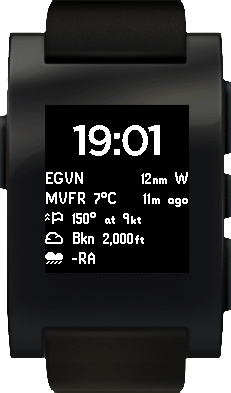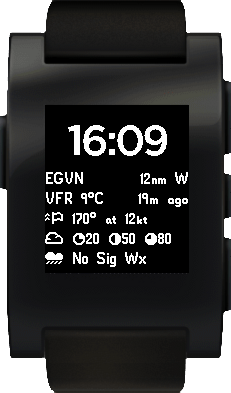Posted 18 Feb 2014 by in Technical

### 6 responses to Pebble Fonts and Metar Watchface

1.Bill McManigle

Glad to know you’re enjoying it! I figured anything that allowed you to program would be the gift that keeps on giving.

2.Holger Dahm

Hi John,

thanks for your script. But I can’t get it working. I tried to convert 2 pfo files into a png.

The first resulted in a

ValueError: total size of new array must be unchanged

during the processing of line

self.bitarray = np.reshape(array[:width*height], (height,width))

The second resulted in a fully black png without any depicted char.

Best regards

Holger Dahm

•John McManigle

Hi Holger,

If you e-mail me (mcmanigle@gmail.com) the PFO files you are trying to convert, I will take a look!

John

3.Sergey Vlasov

The script doesn’t work when using a limited set of characters. This can be set in appinfo.json as “characterRegex”: “[0-9:. ]”.Should be helpful https://github.com/xndcn/pebble-firmware-utils/blob/for-language-pack/extract_codepoints.py

4.norayr

how do you install the font later in the pebble?
assuming i have generated my font, how would i install it?

•John McManigle

It has been a while since I’ve thought about this, but I believe you place the .pfo font file in the resources directory of the app you’re writing, and then the Pebble compilation/packaging script includes it all in your app. The font is then only accessible for use by your app. (There are other resources online for changing Pebble system fonts.)